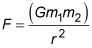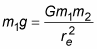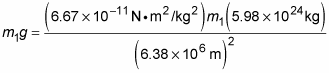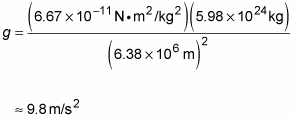##### Physics I For Dummies, 3rd EditionStarting with the physics equation for the force of gravity, you can plug in the mass and radius of the Earth to calculate the force of gravity near the surface of the Earth.

The equation for the force of gravity isand it holds true no matter how far apart two masses are.

The gravitational force between a mass and the Earth is the object’s weight. Mass is considered a measure of an object’s inertia, and its weight is the force exerted on the object in a gravitational field. On the surface of the Earth, the two forces are related by the acceleration due to gravity: Fg = mg. Kilograms and slugs are units of mass; newtons and pounds are units of weight.

You can use Newton’s law of gravitation to get the acceleration due to gravity, g, on the surface of the Earth just by knowing the gravitational constant G, the radius of the Earth, and the mass of the Earth. The force on an object of mass m1 near the surface of the Earth is

F = m1g

This force is provided by gravity between the object and the Earth, according to Newton’s gravity formula, and so you can writeThe radius of the Earth, re, is about 6.38 × 106 meters, and the mass of the Earth is 5.98 × 1024 kilograms. Putting in the numbers, you haveDividing both sides by m1 gives you the acceleration due to gravity:Newton’s law of gravitation gives you the acceleration due to gravity near the surface of the Earth: 9.8 meters/second2.

Of course, you can measure g by letting an apple drop and timing it, but what fun is that when you can calculate it in a roundabout way that requires you to first measure the mass of the Earth?